Function Repository Resource:

# CubicSplineCurve

Generate a cubic B-spline curve that passes through given points

Contributed by: Jan Mangaldan
 ResourceFunction["CubicSplineCurve"][{{x1,y1,…},{x2,y2,…},…}] constructs a cubic interpolating BSplineCurve that passes through the given set of points.

## Details and Options

ResourceFunction["CubicSplineCurve"] returns a BSplineCurve object.
With the setting , ResourceFunction["CubicSplineCurve"] returns a curve that is closed.

## Examples

### Basic Examples (2)

A list of points:

 In:=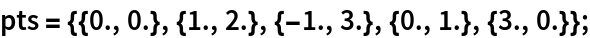Show the points along with an interpolating cubic B-spline:

 In:=Out=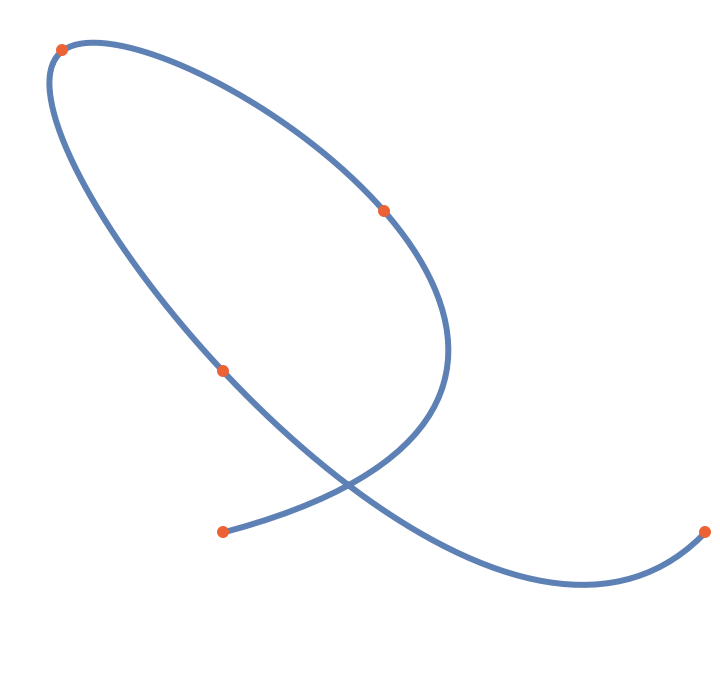### Scope (2)

Choose six points in the plane to be interpolated:

 In:=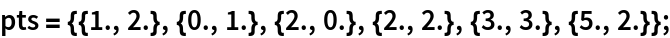Use CubicSplineCurve with Arrow:

 In:=Out=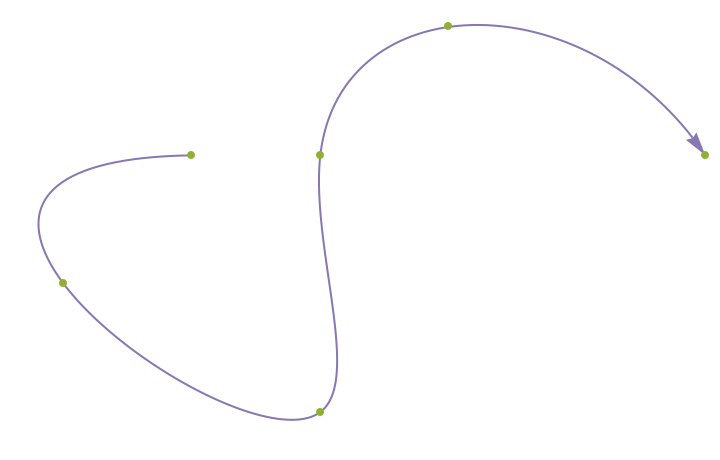Choose 3D points to be interpolated:

 In:=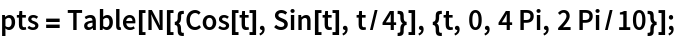Show the points along with an interpolating cubic B-spline:

 In:=Out=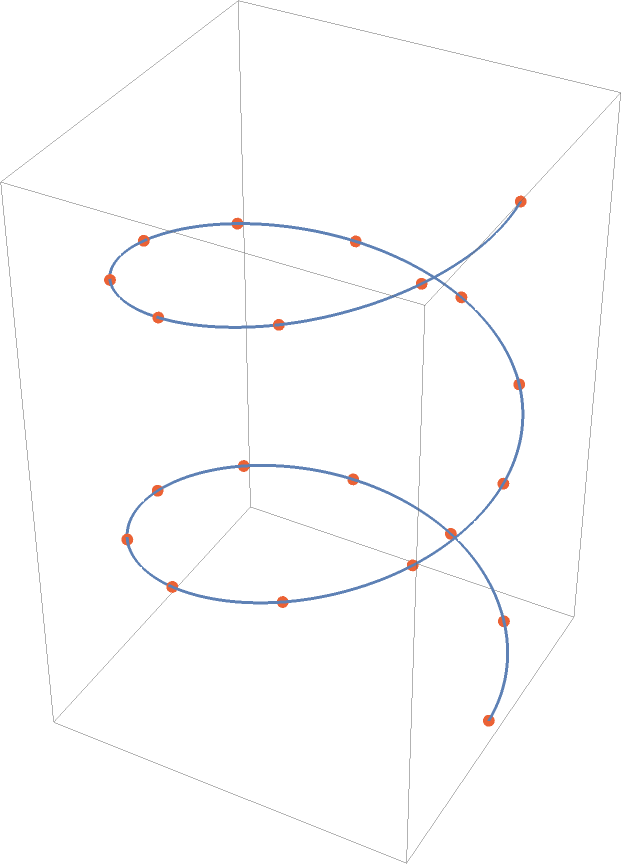Show the interpolating cubic B-spline as a Tube:

 In:=Out=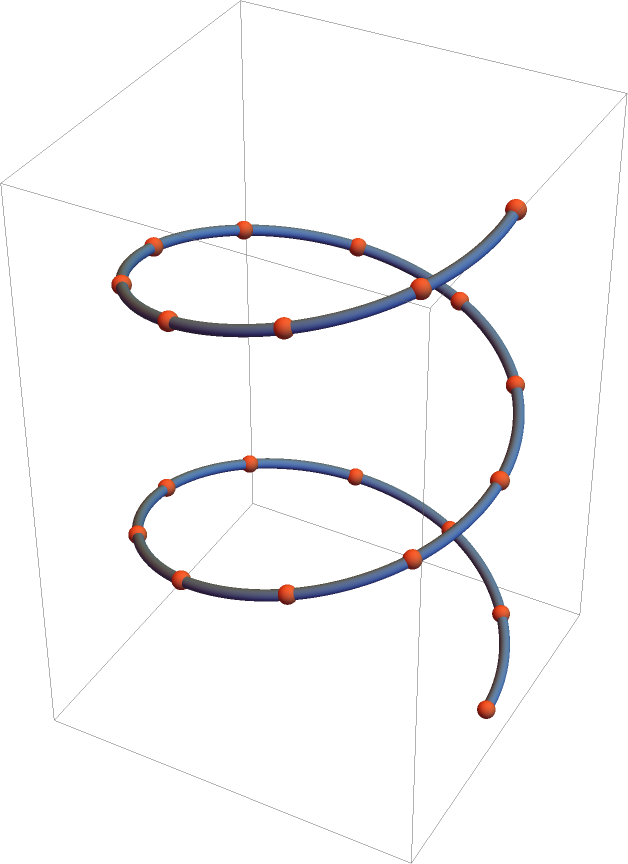### Options (1)

#### SplineClosed (1)

Use to interpolate a set of points with a closed B-spline:

 In:=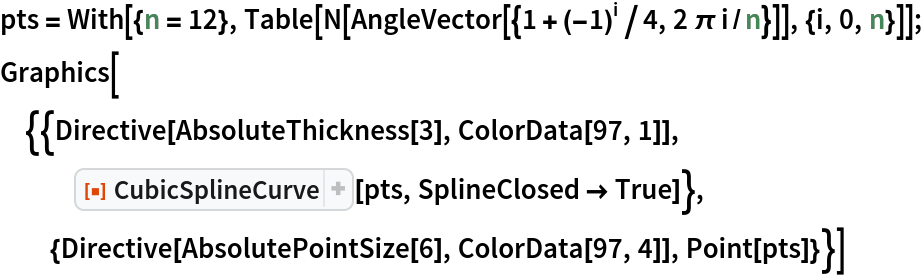Out=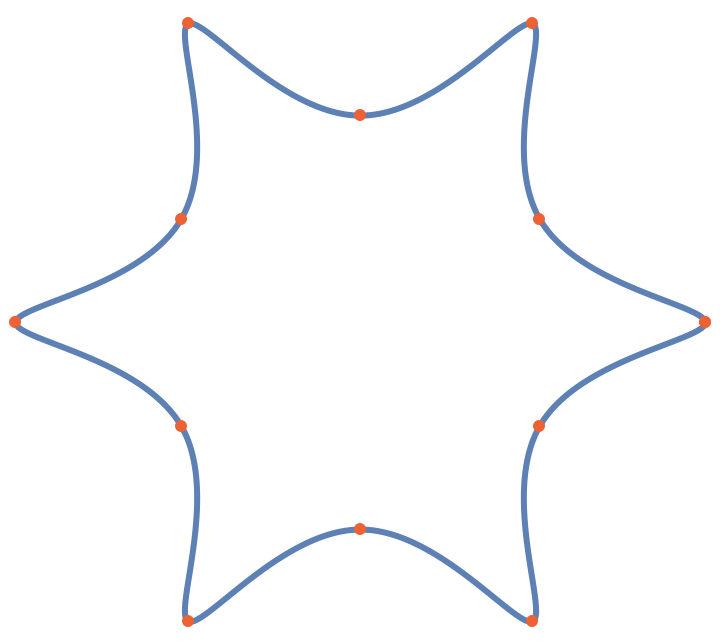### Applications (2)

Convert the result of KnotData into a BSplineCurve:

 In:=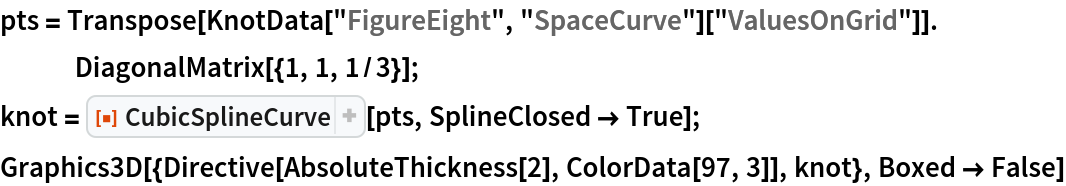Out=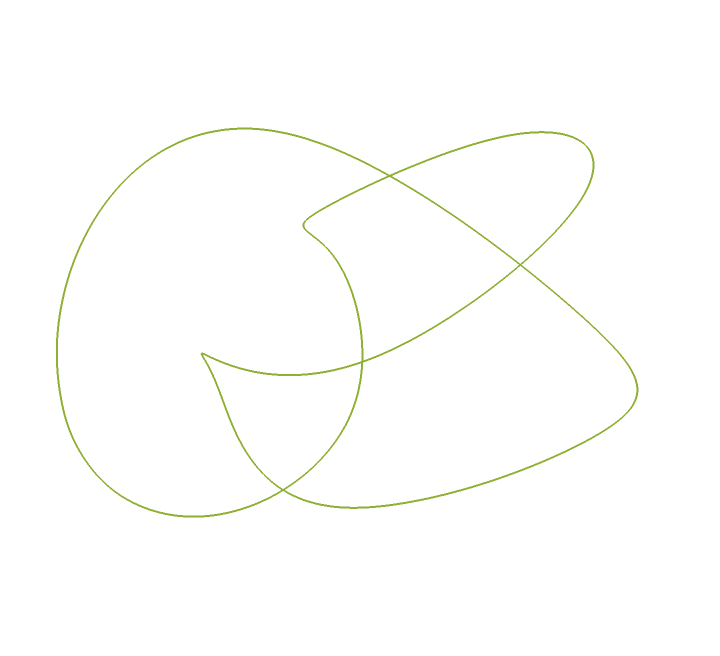Visualize the knot as a Tube:

 In:=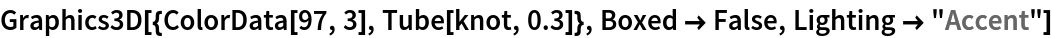Out=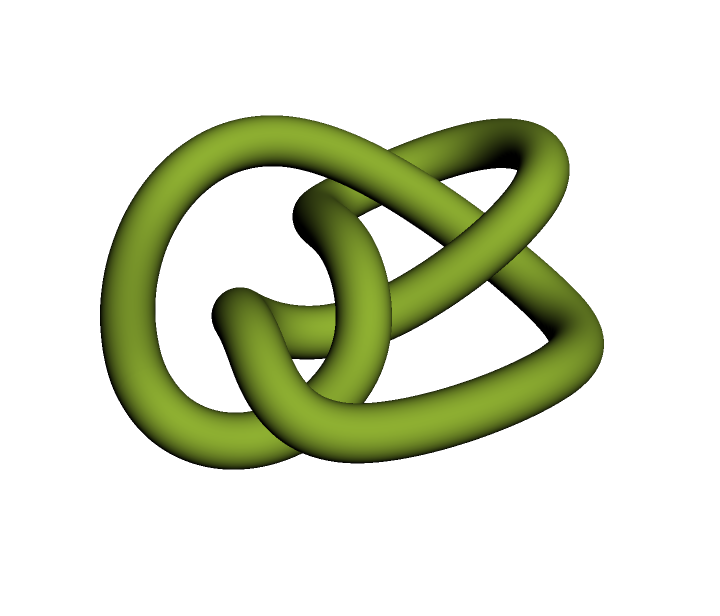## Version History

• 1.0.0 – 03 August 2021